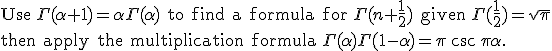# Gamma Function < proving

Hello, I need help proving this:

[URL]http://mathworld.wolfram.com/images/equations/GammaFunction/Inline177.gif[/URL] = [URL]http://mathworld.wolfram.com/images/equations/GammaFunction/Inline179.gif[/URL]

## The Attempt at a Solution

Last edited by a moderator:

## Answers and Replies

Homework Helper
I'm also insterested in a proof of that.

Homework Helper
You should be able to find gamma(1/2) easily enough. A change of variable makes the integral representation a gaussian. Now use gamma(z+1)=z*gamma(z) to find gamma(-1/2). Use that to find gamma(-3/2) etc. Use induction for the general case.

Homework Helper
I'm so dumb, it can be proved by induction, of course.

$$\Gamma\left(\frac{1}{2}-n\right) = \frac{(-1)^{n} 2^{n}}{(2n-1)!!} \sqrt{\pi}$$

Induction says that, if, for a specific n, P(n) is true and you manage to show that P(n+1) is true as well, then P(n) is true for all n from N.

So

$$\Gamma\left(\frac{1}{2}-(n+1)\right) = \Gamma\left(\left(\frac{1}{2}-n\right) -1\right) = \frac{1}{\left(\frac{1}{2}-n\right)-1}}\Gamma\left(\frac{1}{2}-n\right) = \frac{(-1)\cdot 2}{(2n+1)} \Gamma\left(\frac{1}{2}-n\right)$$

Now plug what it's in the hypothesis, and you'll get your answer.

Homework Helper
Now use what I wrote to get a proof of your own for the "sister" equality

$$\Gamma\left(\frac{1}{2}+n\right) = \frac{(2n-1)!!}{2^n}\, \sqrt{\pi}$$

brilliant!! i haven't thought of induction!! but i solved it using another identity:#### Attachments

Homework Helper
You wrote something that came out red as an error message. You can write it with our LaTeX code, or you may put a screenshot of your work.

using the identity:$$\Gamma$$(x) $$\Gamma$$(1-x) = $$\pi$$ / sin(pi *x)